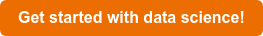# Is this A, or B?

As a follow-up to last week's machine learning tidbit let's look at an example of how we can solve a classification problem using machine learning (on recreational data).

Imagine we're tasked with processing data for an organisation which tracks meets and competitor results in weightlifting. The data itself contains a competitor's bodyweight, sex, age and results.

One of the important things to do here is to create two categories: male and female powerlifting. However, soon enough we notice quite a few blanks in whether the participant was male or female.

Can we predict this?

This leads to the question: can we determine if a person is of category one or two, male or female? In other words; we can use two-class classification to make a prediction. Using the wikipedia page on powerlifting we can see under "classes and categories" that a distinction is made under bodyweight, age and sex.

We can also use the given Wilks* total to get three parameters: bodyweight, age and Wilks total.
(*Wilks is used to compare the strength of powerlifters, ignoring the different weights of the lifters).

The Python data frame we'll use is this:

` df = pd.read_csv(filePath, usecols=['Age', 'BodyweightKg', 'Wilks','Sex']) df.info()`
```<class 'pandas.core.frame.DataFrame'>
RangeIndex: 386414 entries, 0 to 386413
Data columns (total 4 columns):
Sex                    386414 non-null object
Age                    147147 non-null float64
BodyweightKg           384012 non-null float64
Wilks                  362194 non-null float64
dtypes: float64(3), object(1)
memory usage: 11.8+ MB
```

As we have labeled data (and therefor we can use supervised learning) an easy way of doing two-class classification or binary classification is using a logistic regression model.We create a data object, or dataframe, with the four needed columns, do some cleanup and then split it into train and test data. The train data is used to train the model, the test data to test the accuracy.

` # drop null values and create X + ydf = df.dropna(subset=['Age', 'Sex', 'BodyweightKg', 'Wilks'], how='any') X = df[['Age', 'BodyweightKg','Wilks']] y = df[['Sex']] # split dataset X_train, X_test, y_train, y_test = train_test_split(X, y, test_size=0.4, random_state=0) logreg = LogisticRegression() # create logistic regression model logreg.fit(X_train, y_train) # fit training data `

If we then score the model by comparing the test and predicted values we can get an understanding of how well the model performs.

` y_pred = logreg.predict(X_test) # predict test print('Logistic regression accuracy: {:.2f}'.format(logreg.score(X_test, y_test))) # print accuracy`
`Logistic regression accuracy: 0.81`

We can also create a confusion matrix, confusion_matrix(y_test, y_pred), to get a better idea of which values were predicted.From this test we can see that the model correctly predicted 9723 females, 35253 males and incorrectly predicted 10702 entries.

When we're satisfied with a certain accuracy we may implement this model into our process to fill in the blanks in future data.

Get the code here!Topics: data science, python, classification